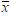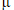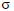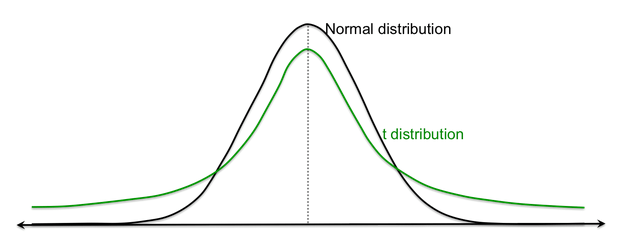PURCHASE A DIGITAL COPY PURCHASE A HARD COPY Lesson 1 Introduction to Statistical Research Methods Lesson 2 Visualizing Data Lesson 3 Central Tendency Lesson 4 Variability Lesson 5 Standardizing Lesson 6 Normal Distribution Lesson 7 Sampling Distributions Lesson 8 Estimation Lesson 9 Hypothesis Testing Lesson 10 t-Tests for Dependent Samples Lesson 11 t-Tests for Independent Samples Lesson 12 Intro to One-Way ANOVA Lesson 13 One-Way ANOVA: Test significance of differences Lesson 14 Correlation Lesson 15 Linear Regression Lesson 16 Chi-Squared Tests Afterward Index

Until now, we’ve assumed we know population parameters and . But often, we don’t. We only have samples from which to draw all our conclusions. In this lesson, you’ll learn a statistical method for concluding whether or not two populations are significantly different. There are two different cases where we can use this statistical test.

Case 1

We have a sample from a certain normally distributed population, and we want to decide if the population mean differs significantly from a certain value.

Examples

1. A growing city is trying to increase tourism to boost its economy. Over the last five years, the average number of new tourists per day has been 378,369. A year after constructing new buildings and tourist attractions, urban planners took a sample of 30 random days throughout the year and found the average number of new visitors to be 432,765. Is this significantly different from the historic population mean of 378,369?
2. This same city has a five-year goal of bringing an average of 500,000 new visitors per day to bring more money into the city and boost its economy. According to this sample, could they have reached their goal of 500,000 new tourists per day?

Case 2

We have two samples from the same population but under different conditions, and we want to decide if these two populations are significantly different.

Example

1. Cell phone designers are deciding between two types of keyboards to use for a new phone they’re developing: the regular QWERTY keyboard, or one arranged in alphabetical order. They want to choose the keyboard that’s easiest to use, so they have a group of xx people type a certain sentence in each phone and record the number of mistakes they make on each. Is the difference significant enough to conclude that one keyboard is easier to use than the other?

Let’s go through Case 1 first. Our sample, with mean, comes from a population with meanand standard deviation. We knowand s, but not. We have to use our sample statistics to decide ifis significantly different from a particular value ().Before, when we knew the parameters of the population from which the sample was drawn, we could find where that sample mean fell on the sampling distribution by calculating the  standard error () and the z-statistic for that sample ().

But now the case is slightly different: we’re usingto approximatein order to compareto, and we’re using the standard deviation of our sample (s) to approximate. If we use s to approximateandto approximate, we end up with a new type of distribution that approximates the normal population distribution, called a t-distribution.Since the t-distribution is based on approximations, it has more error than the normal distribution, visualized by the thicker tails.

To continue learning, purchase Street-Smart Stats: A Friendly Introduction to Statistical Research Methods.